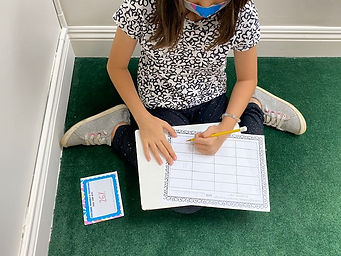## Ms. Dawn

### Target 1​

###### Lesson Type:

Continuation

Number Operation

:

Fractional Numbers

Convert between fractions, decimals, and percentages.

###### 1:

Describe the relationship between fractions, decimals, and percentages.

###### 2:

Convert fractions to decimals and decimals to fractions.

###### 3:

Identify the fractional equivalent of a percent.

4th

###### Vocabulary:

Fraction, Decimal, Percent, Covert, Equivalent

Activities:

1. Reviewed the idea of converting decimals to fractions and fractions to decimals. Students refreshed their memory about those concepts first.

2. After the review, we discussed percentage and the meaning. We talked about basic percentages of the denominator being 10 or 100. Then, we discussed different strategies on how to turn fractions into percents. We talked about how to break down each part of the whole and figure how much it is worth out of a hundred.

3. I had put a variety of task cards around the room dealing with converting fractions to decimals, fractions to percents, decimals to fractions, and decimals to percents.### Home Exploration

###### Guiding Questions:## Absent Students:

### Target 2

:

###### 1:

Convert improper fractions to mixed numbers (and vice versa), understanding that both have “wholes” as a part of their quantity.

###### 2:

Understand the equivalent relationship between mixed numbers and improper fractions.

4th

###### Vocabulary:

Mixed Number, Improper Fraction, Convert, Equivalent, Whole, Parts of a whole, Numerator, Denominator

Activities:

1. We discussed the terms "Mixed Number" and "Improper Fraction." Students realized that it is the same fraction, just written and stated differently.

2. Students learned strategies on how to convert a mixed number to an improper fraction and vice versa. They used the strategy like the shape of a "V" sideways. For converting the mixed number to an improper fraction, they learned to write the sideways "V," then write a multiplication sign on the bottom and a plus sign on the top part of the "V." It helped them process the steps, which made it easier. Then for the improper fraction to a mixed number, they also made a sidways "V." They got into the routine of asking themselves, how many times can the denominator go into the numerator without going over. Then, they would write that number above by the numerator and then subtract it. It became a habit to draw the "V" and write the symbols.

After a few practice problems on their whiteboard, we did a challenge with a variety of problems. It was a timed challenge. Students showed their understanding of coverting mixed numbers to improper fractions and vice versa.### Home Exploration

###### Guiding Questions:### Target 3

:

###### Vocabulary:

Activities:### Home Exploration